# A proton moving at 7.00 x 106 m/s through a magnetic field of magnitude 2.6 T...

A proton moving at 7.00 x 106 m/s through a magnetic field of magnitude 2.6 T experiences magnetic force of magnitude 6.9x10-13 N. What is the angle between the proton's velocity and the field?

6.2°

76.3°

13.7°

44.9°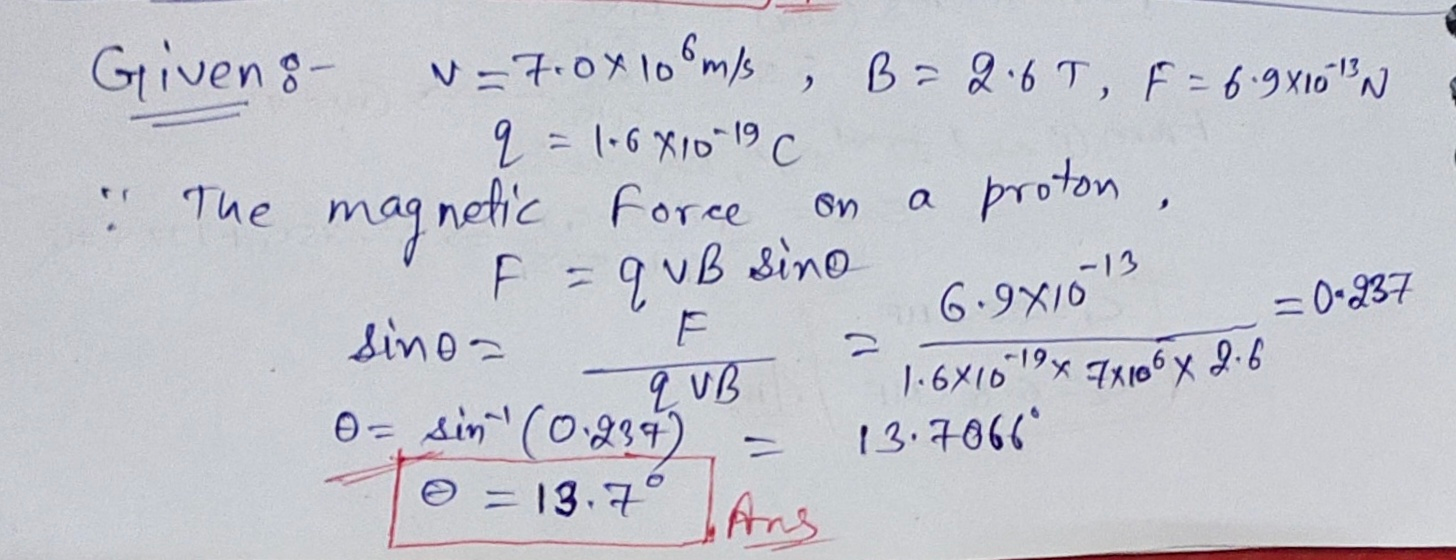13.7°

#### Earn Coin

Coins can be redeemed for fabulous gifts.

Similar Homework Help Questions
• ### Question 13 3 pts A proton moving at 7x109 m/s through a magnetic field of magnitude...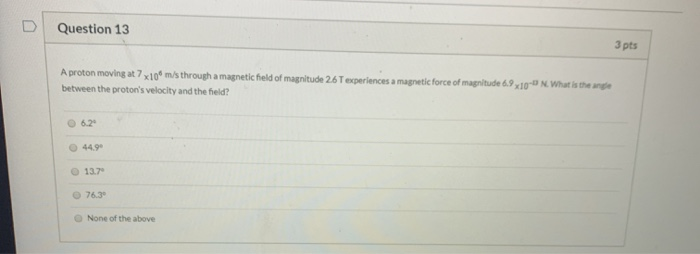Question 13 3 pts A proton moving at 7x109 m/s through a magnetic field of magnitude 26 T experiences a magnetic force of magnitude 6.9x10- N What is the angle between the proton's velocity and the field? O 6.2 44.99 13.7 76.39 None of the above

• ### A proton moving at 4.50 106 m/s through a magnetic field of magnitude 1.80 T experiences...

A proton moving at 4.50 106 m/s through a magnetic field of magnitude 1.80 T experiences a magnetic force of magnitude 7.80 10-13 N. What is the angle between the proton's velocity and the field? (Enter both possible answers from smallest to largest.) a. in ° b. in °

• ### A proton moving at 6.60 106 m/s through a magnetic field of magnitude 1.78 T experiences...

A proton moving at 6.60 106 m/s through a magnetic field of magnitude 1.78 T experiences a magnetic force of magnitude 7.20 10-13 N. What is the angle between the proton's velocity and the field? (Enter both possible answers from smallest to largest. Enter only positive values between 0 and 360.)

• ### A proton moving at 5.80 106 m/s through a magnetic field of magnitude 1.78 T experiences...

A proton moving at 5.80 106 m/s through a magnetic field of magnitude 1.78 T experiences a magnetic force of magnitude 8.00 10-13 N. What is the angle between the proton's velocity and the field? (Enter both possible answers from smallest to largest. Enter only positive values between 0 and 360.) _________ smaller value ° __________ larger value °

• ### A proton moving at 5.80 106 m/s through a magnetic field of magnitude 1.80 T experiences...

A proton moving at 5.80 106 m/s through a magnetic field of magnitude 1.80 T experiences a magnetic force of magnitude 8.00 10-13 N. What is the angle between the proton's velocity and the field? (Enter both possible answers from smallest to largest. Enter only positive values between 0 and 360.) smaller value ° larger value °

• ### A proton moving at 3.50 times 10^6 m/s through a magnetic field of magnitude 1.78 T...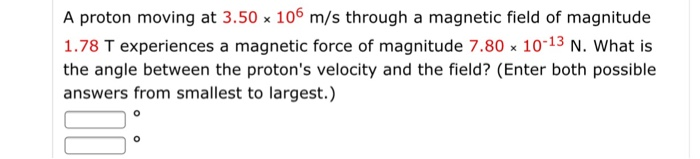A proton moving at 3.50 times 10^6 m/s through a magnetic field of magnitude 1.78 T experiences a magnetic force of magnitude 7.80 times 10^-13 N. What is the angle between the proton's velocity and the field? (Enter both possible answers from smallest to largest.)

• ### An electron moving at 4.5x106 m/s through a magnetic field and experiences a magnetic force of...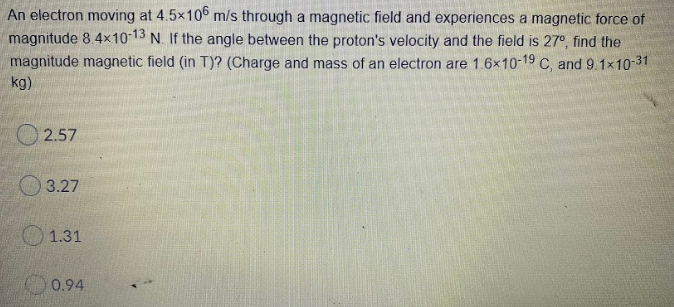An electron moving at 4.5x106 m/s through a magnetic field and experiences a magnetic force of magnitude 8.4x10-13 N. If the angle between the proton's velocity and the field is 27°, find the magnitude magnetic field (in T)? (Charge and mass of an electron are 1.6x10-19 C, and 9.1x10-31 kg) 2.57 3.27 1.31 0.94

• ### A proton moving at 3500 m/s in a 2.35 T magnetic field experiences a magnetic force...

A proton moving at 3500 m/s in a 2.35 T magnetic field experiences a magnetic force of 1.20×10-15 N. What angle does the velocity of the electron make with the magnetic field? State all possible answers.

• ### 030 (part 1 of 2) 10.0 points A proton in a cyclotron is moving with a...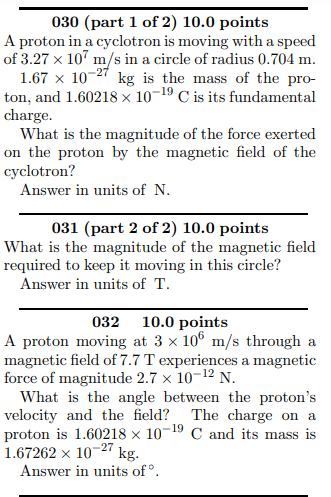030 (part 1 of 2) 10.0 points A proton in a cyclotron is moving with a speed of 3.27 x 10 m/s in a circle of radius 0.704 m. 1.67 x 10-27 kg is the mass of the pro- ton, and 1.60218 x 10-19 C is its fundamental charge. What is the magnitude of the force exerted on the proton by the magnetic field of the cyclotron? Answer in units of N. 031 (part 2 of 2) 10.0 points What...

• ### A proton moving at 8.2×106 m/s enters a magnetic field of 0.38 Tesla, with the velocity...

A proton moving at 8.2×106 m/s enters a magnetic field of 0.38 Tesla, with the velocity vector at 90o to the magnetic field direction. Calculate the magnitude of the force exerted on the particle.